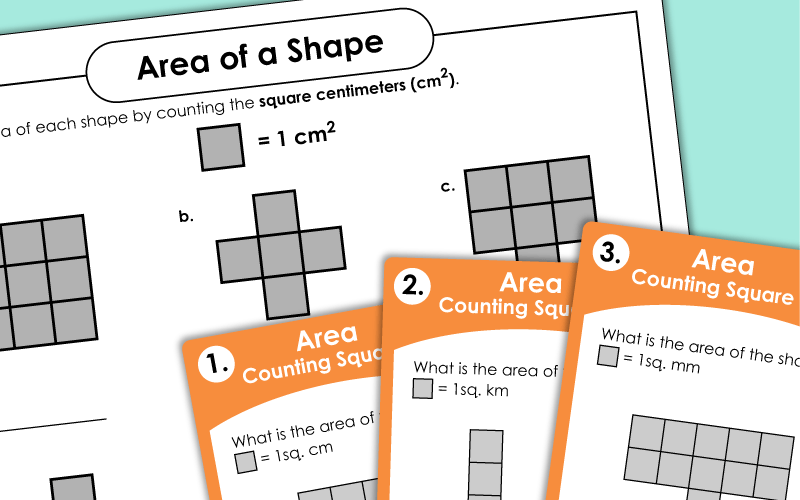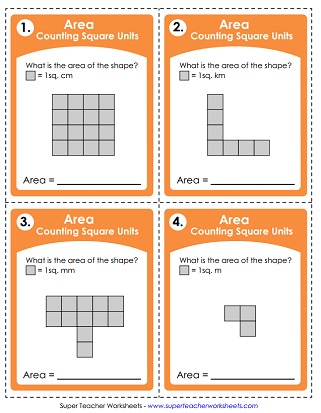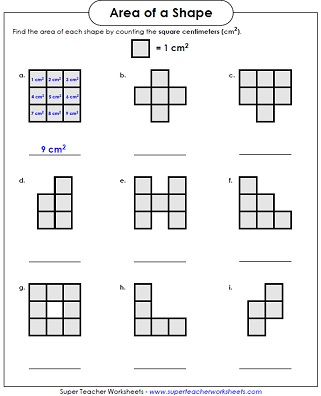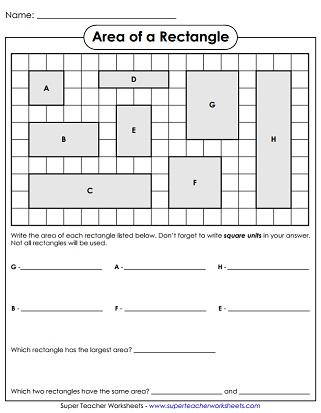# Area - Counting Square Units

These are very basic level area worksheets. Students count the square units to find the area of the shapes. Worksheets include non-standard and standard units.## Worksheets

Count the squares to determine the number of square units each shape has.
Find the area of each shape by counting the square units.
This type is a little harder because the square grid is not drawn inside the shapes.
Part 1: Find the area of each shape by counting the square units. Part 2: Then draw squares to find the number of square units for each shape. Part 3: Complete the short answer question.
Count the centimeters to find the perimeter and area of each shape.
Find the perimeter and area of each rectangle.  Includes examples.
On the grid paper shown, draw a rectangle with an area of 20 square units. Then draw a square that is 4 square units in size. Then draw another square that is 9 times as large.

Use these 30 cards for classroom scavenger hunts, whole group instruction, or learning centers. Count the square millimeters, centimeters, meters, and kilometers to determine the areas of the polygons.
These "counting square units" task cards are slightly harder than the set listed above.

## Area Games

In this 2 player game, students take turns rolling pairs of dice. They use crayons and graph paper to make rectangles with the number of square units rolled.
Students draw their name on the included graph paper. Then they write the area (in square cm) of each letter.
Area of Rectangles

Multiply the length times width to find the areas of rectangles and squares.

More Area Worksheets

Find more advanced geometry worksheets on calculating the area. Includes printables on finding areas of rectangles, triangles, parallelograms, trapezoids, and circles.

Perimeter Worksheets

Calculate the perimeter of the polygons by adding the lengths of the sides.

Geometry Worksheets (Index Page)

Geometry topics on Super Teacher include angles, symmetry, polygons, solid shapes, plotting points, and more.

## Sample Images of Our PrintablesMy Account
Site Information